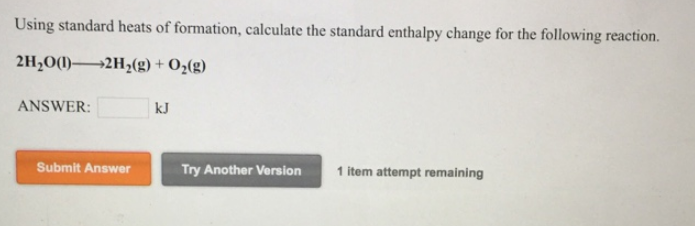# Problem: Using standard heats of formation, calculate the standard enthalpy change for the following reaction. 2H2O (l) → 2H2 (g) + O2 (g)

###### FREE Expert Solution
79% (91 ratings)###### Problem Details

Using standard heats of formation, calculate the standard enthalpy change for the following reaction.

2H2O (l) → 2H(g) + O2 (g)